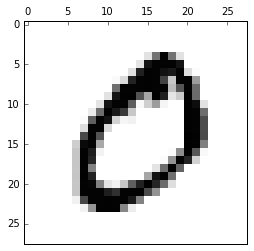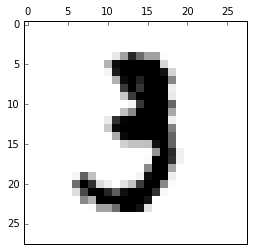Credits: Forked from deep-learning-keras-tensorflow by Valerio Maggio

# ConvNet HandsOn with Keras¶

## Problem Definition¶

Recognize handwritten digits

## Data¶

The MNIST database (link) has a database of handwritten digits.

The training set has $60,000$ samples. The test set has $10,000$ samples.

The digits are size-normalized and centered in a fixed-size image.

The data page has description on how the data was collected. It also has reports the benchmark of various algorithms on the test dataset.

The data is available in the repo's data folder. Let's load that using the keras library.

For now, let's load the data and see how it looks.

In :
import numpy as np
import keras
from keras.datasets import mnist

Using Theano backend.
Using gpu device 0: GeForce GTX 760 (CNMeM is enabled with initial size: 90.0% of memory, cuDNN 4007)

In :
!mkdir -p \$HOME/.keras/datasets/euroscipy_2016_dl-keras/data/

In :
# Set the full path to mnist.pkl.gz
path_to_dataset = "euroscipy_2016_dl-keras/data/mnist.pkl.gz"

In :
# Load the datasets
(X_train, y_train), (X_test, y_test) = mnist.load_data(path_to_dataset)

Downloading data from https://s3.amazonaws.com/img-datasets/mnist.pkl.gz
15024128/15296311 [============================>.] - ETA: 0s

# Basic data analysis on the dataset¶

In :
# What is the type of X_train?

In :
# What is the type of y_train?

In :
# Find number of observations in training data

In :
# Find number of observations in test data

In :
# Display first 2 records of X_train

Out:
array([[[0, 0, 0, ..., 0, 0, 0],
[0, 0, 0, ..., 0, 0, 0],
[0, 0, 0, ..., 0, 0, 0],
...,
[0, 0, 0, ..., 0, 0, 0],
[0, 0, 0, ..., 0, 0, 0],
[0, 0, 0, ..., 0, 0, 0]],

[[0, 0, 0, ..., 0, 0, 0],
[0, 0, 0, ..., 0, 0, 0],
[0, 0, 0, ..., 0, 0, 0],
...,
[0, 0, 0, ..., 0, 0, 0],
[0, 0, 0, ..., 0, 0, 0],
[0, 0, 0, ..., 0, 0, 0]]], dtype=uint8)
In :
# Display the first 10 records of y_train

Out:
array([5, 0, 4, 1, 9, 2, 1, 3, 1, 4], dtype=uint8)
In :
# Find the number of observations for each digit in the y_train dataset

Out:
(array([0, 1, 2, 3, 4, 5, 6, 7, 8, 9], dtype=uint8),
array([5923, 6742, 5958, 6131, 5842, 5421, 5918, 6265, 5851, 5949]))
In :
# Find the number of observations for each digit in the y_test dataset

Out:
(array([0, 1, 2, 3, 4, 5, 6, 7, 8, 9], dtype=uint8),
array([ 980, 1135, 1032, 1010,  982,  892,  958, 1028,  974, 1009]))
In :
# What is the dimension of X_train?. What does that mean?

Out:
(60000, 28, 28)

### Display Images¶

Let's now display some of the images and see how they look

We will be using matplotlib library for displaying the image

In :
from matplotlib import pyplot
import matplotlib as mpl
%matplotlib inline

In :
# Displaying the first training data

In :
fig = pyplot.figure()
imgplot = ax.imshow(X_train, cmap=mpl.cm.Greys)
imgplot.set_interpolation('nearest')
ax.xaxis.set_ticks_position('top')
ax.yaxis.set_ticks_position('left')
pyplot.show()In [ ]:
# Let's now display the 11th record

In :